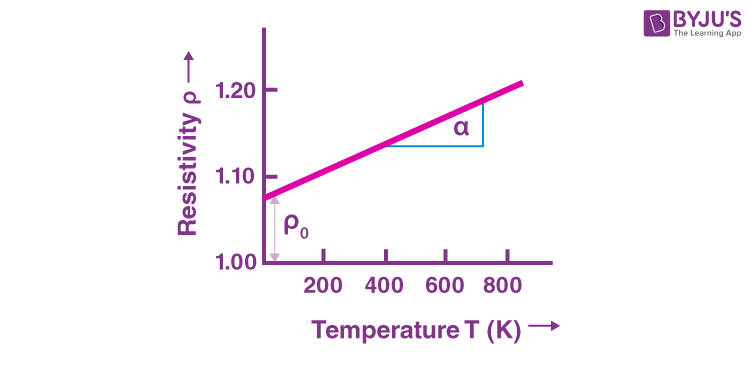# The Temperature Dependence Of Resistance

Resistance is fundamentally the ability of the material to restrict the passage of electric current. Thus, just as material properties such as density, size, magnetisation etc. Change with temperature, so does resistance. Resistance is really a bulk property; the resistivity, on the other hand, is a material property. We shall talk about the temperature dependence of resistivity in this article.

## Resistivity of Metals

The resistivity (ρ) of metals is dependent on the relaxation time (τ) of the free electrons in the metal as

$$\begin{array}{l}ρ ∝ \frac {1}{τ}\end{array}$$

(See our article on drift velocity)

The relaxation time is dependent on the mean free path (λ) and the root-mean-square speed (vrms) of the electron as:

$$\begin{array}{l}τ = \frac{v_{rms}}{λ}\end{array}$$

$$\begin{array}{l}ρ ∝ \frac {1}{τ} ∝ \frac{λ}{v_rms} \end{array}$$

Since the root-mean-square speed increases with temperature and means free path, the resistivity and hence the resistance of the metal also rises.

This is mostly linear and is given as:

$$\begin{array}{l} ρ_t = ρ_0 (1~+~αt)\end{array}$$

Where, ρt  is the resistivity at t degrees centigrade and ρ0  is the resistivity at 0 degrees Celsius.### Resistivity of Semiconductors

The number of charge carriers in semiconductors is lesser than that of insulators. Semiconductors like Silicon and Germanium contains lesser charges than those of metals but has more charges than in insulators. These carriers are generated by the thermal breaking of bonds. Thus, as the temperature rises, more number of covalent bonds break, releasing more electrons which lowers the resistivity rapidly. This temperature dependence is usually exponential which makes semiconductors very useful in electronic circuits detecting small changes in temperature.Learn to apply your conceptual knowledge to real-world applications with us at BYJU’S!

## Frequently Asked Questions – FAQs

Q1

### What is resistance?

Resistance is the ability of the material to restrict the flow of electric current.
Q2

True.
Q3

### What is the unit of electrical resistance?

The unit of electrical resistance is ohms.
Q4

### List two factors on which resistance depends.

Resistance depends upon:
• The cross-sectional area of the conductor.
• The temperature of the conducting material.
• Q5

### What is the resistivity of the air?

The resistivity of air is 2.30 × 1016
Test your knowledge on Temperature Dependence Resistance